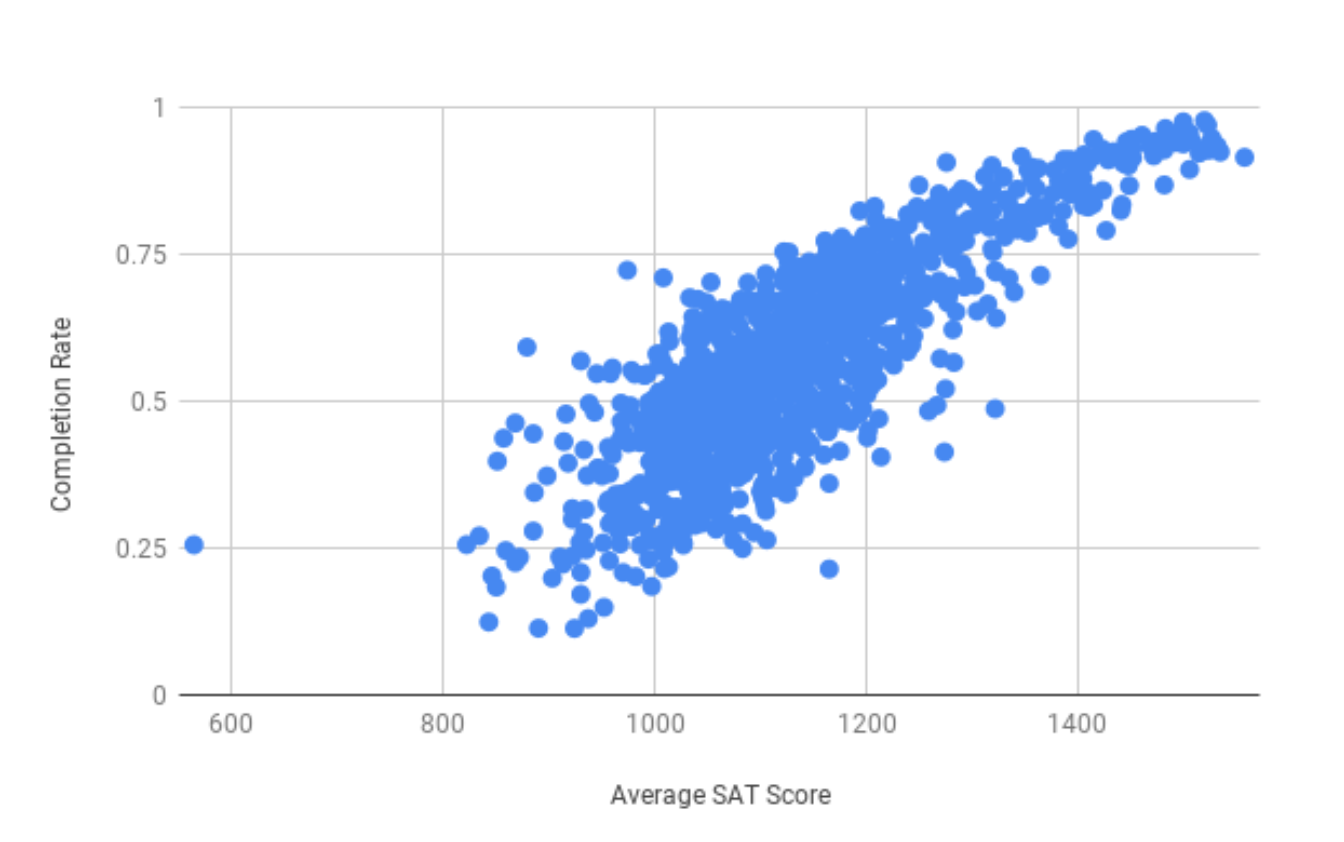# Example: Correlation and College Data¶

Correlation is also used to find which variable might be useful in predicting another variable. For example, consider a dataset that contains the following variables.

• shoe size

• age

• salary

• height

Using this dataset, you want to be able to predict the height of a person, using only one other variable: shoe size, age, or salary. To get the best prediction, you would choose whichever variable has the strongest relationship with height. Correlation is the most standard metric for quantifying how strong a relationship is between two variables.

Now, you will apply the concepts you’ve learned so far to help identify relationships in a dataset about colleges and universities across the USA. For each school, the dataset has information about the following.

• The state where the school is located.

• The type of institution: public, private non-profit, or private for-profit.

• The median SAT scores for reading, math, and overall.

• The average net tuition, i.e. the mean of the amount paid by the student after applying scholarships and other forms of financial aid.

• The percentage of enrolled students receiving Pell Grants.

• The percentage of enrolled students receiving federal loans.

• The median earnings for employed students 10 years after first enrolling.

• The median debt of graduates of the school.

• The completion rate, i.e. the percentage of full-time, first-time students who graduate within 6 years.

Suppose you want to know which variable has the strongest relationship with completion rate. In this dataset, completion is defined as the percentage of full time students who graduate within 6 years without transferring to another institution. In other words, “completion” is used as a proxy for graduation rate. Using the CORREL function, you can calculate the correlation between completion rate and the other variables.

Out of all the explanatory variables, it is “SAT average” that has the closest correlation to -1 or 1 with completion rate, at r = 0.84. Looking at the scatter plot, SAT average and completion rate have a strong, positive, linear relationship.Q-1: Use the scatter plot to estimate the completion rate at a school with an average SAT score of 1200.

Q-2: Use the scatter plot to estimate the completion rate at a school with an average SAT score of 1400.

Q-3: Which estimate do you think was more accurate? Why?

Q-4: Which variable has the strongest relationship with median debt?

Q-5: What two variables have a correlation coefficient closest to -1?